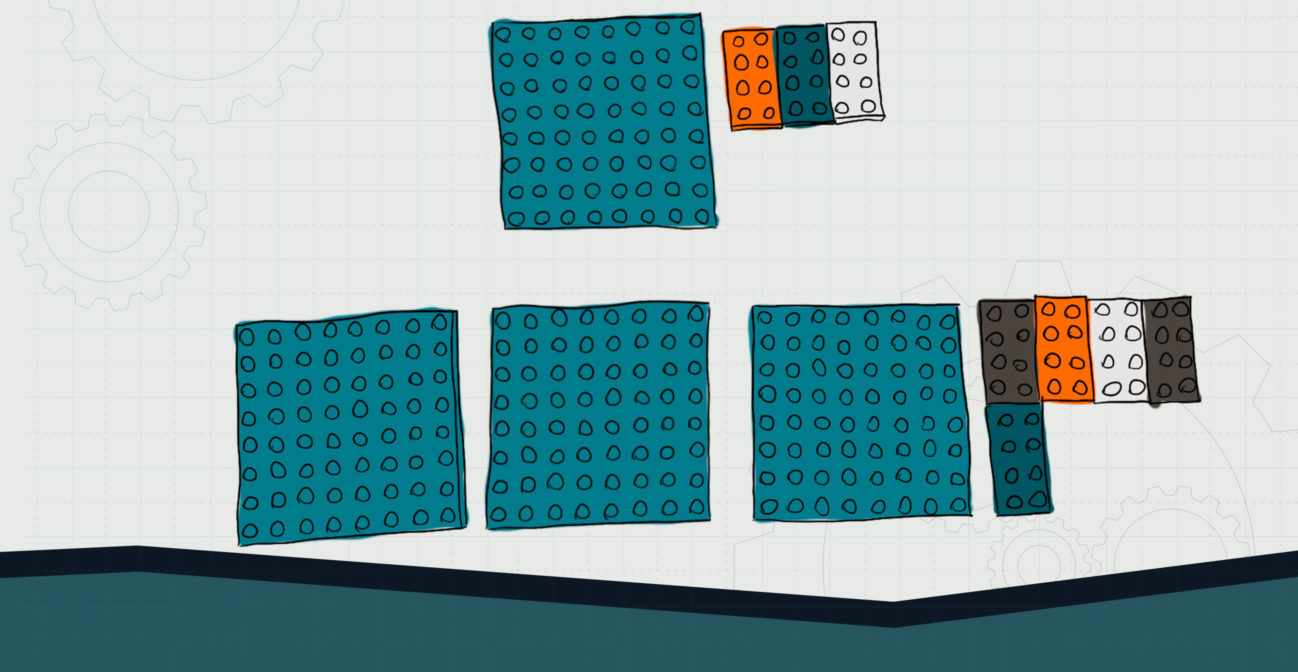# Mixed Bricks

## Explore mixed fractions and addition through bricks.Objective:
Child will be able to add mixed fractions by physically regrouping.

Essential Question(s):
How do you add mixed fractions?

Special Materials:
Pencil and paper, dice for choosing numbers

Bricks Required:
Plates that are divisors of each other (i.e., 8x8s and 2x4s)

Project Structure

Engage/Explain:

2. Ask what child thinks about adding mixed fractions. How might they do it? How would they account for the whole numbers and fractions?
1. Child may come up with the idea that they need to add the whole numbers and fractions separately.
2. Child may come up with the idea that they may need to regroup the fractions if the fractions add up to more than a whole.

Explore:

1. Give child a die, a stack of 8×8 plates, and a handful of smaller plates.
1. Have child practice adding mixed fractions with 8ths as the denominator.
2. Ask child to use the die to roll numbers to add. First roll is the whole number, second roll is the numerator. Repeat for the whole number and numerator for the second portion of the equation (be writing down the equation). For example, if child rolls 6, 3, 2, 5, then the equation will be 6 ⅜ + 2 ⅝.
3. Child then uses the 8×8 plates for the whole numbers and the 2×4 plates for the fractions. The 2x4s can be rearranged to make 8×8 squares.